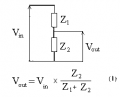Difference in circuitry of low and high pass filters

Juventus1994

Joined Nov 14, 2020
1
Hello folks,

I am an electronic student currently. in class we are learning about low and high pass filters.
I understand that in a low pass filters, the resistor is first and then the capacitor.
In a high pass filter, it is the capacitor first and then the resistor.
I'm trying to understand why there is this difference in circuitry.

I understand that low pass allow only frequencies to a certain point (frequency cut off) to pass through, and high pass only let certain high frequencies pass through but not low frequencies. I also understand that the higher the frequency, the low reactance (resistance) the capacitor has and vice versa. despite knowing this when I look at the circuit and have no idea why low pass has resistor first and then capacitor and vise versa for high pass.

If anyone can help me understand that would be much appreciated. I don't know if it makes a difference, bur we are using AC as the power source.

Audioguru again

Joined Oct 21, 2019
4,096
Why doesn't your teacher teach this simple stuff??

The resistor and capacitor make a voltage divider:
1) The lowpass filter capacitor conducts high frequencies to ground through the capacitor. The resistor passes low frequencies to the output.
2) The highpass filter capacitor blocks low frequencies but passes high frequencies to the output.

Delta Prime

Joined Nov 15, 2019
1,213
Hello thereBoy, I could be totally wrong I hope but... And I'm not trying to beat you up,I believe electronic principles and circuits maybe be beyond on your scope and only add to confusion. Your instructor put the cart way ahead of the horse. I admire your enthusiasmMrChips

Joined Oct 2, 2009
24,998
An RC filter is a voltage divider as AG says.Vout is a fraction of the input voltage Vin.

For an RC filter, we replace either Z1 or Z2 with a component (in this case capacitor) whose impedance Z is a function of frequency. When we do that, examine how Vout is also a function of frequency.

To put it simply, Zc is infinite at 0Hz and decreases with frequency. Examine Vout when Zc is in position Z1 and then in position Z2 at different frequencies.

(BTW, this is a simplistic model. The correct mathematical model requires you to apply complex arithmetic which hopefully you will encounter soon in your courses.)

ericgibbs

Joined Jan 29, 2010
14,664
hi 1994,
Welcome to AAC.

Look at this simple plot of Capacitive reactance [ impedance ] versus frequency.
As you can see the impedance of a capacitance decreases as the frequency of the applied series sine wave increases.

Look at the image of the two Res/Cap circuits, consider what happens to the level of the output voltage, due to changes in the Capacitive impedance, as the input frequency is varied.

E

LvW

Joined Jun 13, 2013
1,353
I understand that in a low pass filters, the resistor is first and then the capacitor.
In a high pass filter, it is the capacitor first and then the resistor.
I'm trying to understand why there is this difference in circuitry.
I do not want to confuse you - but you should keep in mind, that those RC-filter stages you have mentioned are just one of several alternatives for realizing filters.
The simple voltage divider principles with one capactive resistance (impedance) can also be realized with an inductor and one resistor. Example: An R-C lowpass can be replaced by an L-R lowpass.
All these simple stages are so called "first-order" filters (because they contain one frequency-dependent part only).
There are higher-order stages with better filter capabilities...but this question probably is outside of your problem presently...

MrAl

Joined Jun 17, 2014
8,606
Hello folks,

I am an electronic student currently. in class we are learning about low and high pass filters.
I understand that in a low pass filters, the resistor is first and then the capacitor.
In a high pass filter, it is the capacitor first and then the resistor.
I'm trying to understand why there is this difference in circuitry.

I understand that low pass allow only frequencies to a certain point (frequency cut off) to pass through, and high pass only let certain high frequencies pass through but not low frequencies. I also understand that the higher the frequency, the low reactance (resistance) the capacitor has and vice versa. despite knowing this when I look at the circuit and have no idea why low pass has resistor first and then capacitor and vise versa for high pass.

If anyone can help me understand that would be much appreciated. I don't know if it makes a difference, bur we are using AC as the power source.

The most basic property of a capacitor is that of its ability to pass an AC current increases as the frequency goes higher. If one end of the cap is grounded it is going to pass higher frequency to ground where they will never make it to the output thus allowing only lower frequencies to make it out, but if it is in series with the signal then as the frequency goes up more of the signal will get to the output.

As others have suggested, do a careful study of a resistive voltage divider and see what happens when measure the output signal after you make one of the resistors small and one large, then swap the two and see what happens to the output signal then. When the series resistor is small you will see one response, and when the lower resistor is small you see a different amplitude.

That suggests that depending on where the cap is placed in the actual filter the output response changes accordingly.